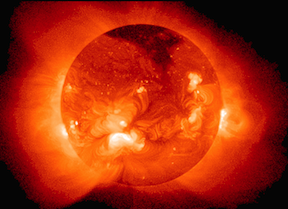# Multiple Dimensions - Calculate The Mass Of The SunAs humans haven't been able to reach the sun, we have to work with mathematical models of the interior of the sun based on observations that are made from afar. The standard model of the sun assumes that the density of heated gas ( in grams per cm$^3$) throughout the interior follows the best-fit formula $D(x) = 519x^4 - 1630 x^3 + 1844 x^2 - 889 x + 155,$ where $x=0$ represents the core, and $x = 1$ represents the surface. Given that the physical radius of the sun is $6.90 \times 10^{10}$ centimeters, what is the total mass of the sun (in kg)?

This problem is part of Calvin's set Fun In Multiple Dimensions.

Hint: We are integrating along the radius. What is the corresponding area element?

×